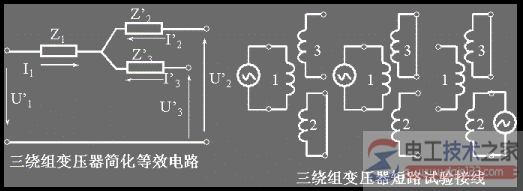# 三绕组变压器的等效电路特性的计算方法

## 三绕组变压器等效电路特性的计算方法

3个变比：

k12= N1/N2 ≈ U1 / U20

k13= N1/N3 ≈ U1 / U1

k23= N2/N3 ≈ U20 / U30

I1N1+I2N2+I3N3=0

I1+I2/k12+I3/k13=0

I1+I2'+I3'=0

Zk12=Rk12+jXk12= (R1+R2') + j(X1+X2')

Zk13=Rk13+jXk13=(R1+R3')+j(X1+X3')

Zk23'=Rk23'+jXk23'=(R2'+R3')+j(X2'+X3')

R1=1/2(Rk12+Rk13-Rk23')

X1=1/2 (Xk12+Xk13-Xk23')

R2'=1/2(Rk12+Rk23'-Rk13)

X2'=1/2 (Xk12+Xk23'-Xk13)

R3'=1/2(Rk13+Rk23'-Rk12)

X3'=1/2(Xk13+Xk23'-Xk12)`相关文章`